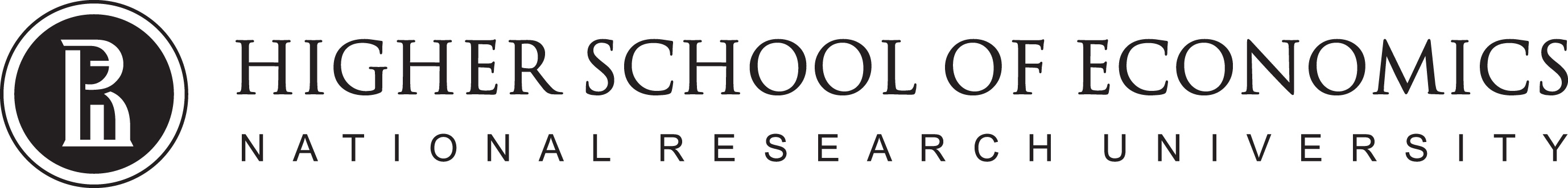# Data Analysis

## Linear classification. Logistic Regression1

1. Some materials are taken from machine learning course of Victor Kitov

# Let's recall previous lecture¶

• Linear regression
• linear dependence between target features and predictors
$$f(x_{n}, \beta) = \hat{y}_{n} = \beta_0 + \beta_1x_{n}^1 + \dots$$
• Optimize Ordinary Least Squares
• Solution can be found
• analytically

# Regularization & restrictions¶

## Intuition¶[Andrew's Ng Machine Learning Class - Stanford]

## Regularization¶

• Regularization - technique that reduces model complexity to prevent overfitting
• Desired outcomes:
• train loss goes up
• test loss goes down
• Have we already seen it?

## Regularization¶

• Insert additional requirement for regularizer $R(\beta)$ to be small: $$\sum_{n=1}^{N}\left(x_{n}^{T}\beta-y_{n}\right)^{2}+\lambda R(\beta)\to\min_{\beta}$$
• $\lambda>0$ - hyperparameter.
• $R(\beta)$ penalizes complexity of models. $$\begin{array}{ll} R(\beta)=||\beta||_{1} & \mbox{Lasso regression}\\ R(\beta)=||\beta||_{2}^{2} & \text{Ridge regression} \end{array}$$
• Not only accuracy matters for the solution but also model simplicity!
• $\lambda$ controls complexity of the model:\uparrow\lambda\Leftrightarrow\text{complexity}$$\downarrow. ## Comments¶ • Dependency of \beta from \lambda for ridge (A) and LASSO (B):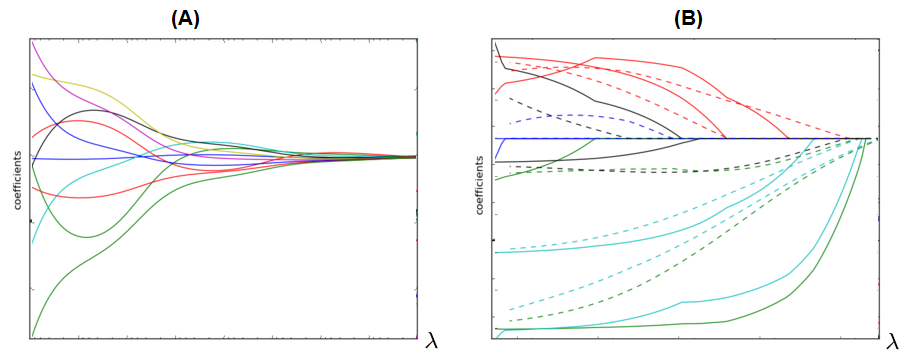• LASSO can be used for automatic feature selection. • \lambda is usually found using cross-validation on exponential grid, e.g. [10^{-6},10^{-5},...10^{5},10^{6}]. • It's always recommended to use regularization because • it gives smooth control over model complexity. • reduces ambiguity for multiple solutions case.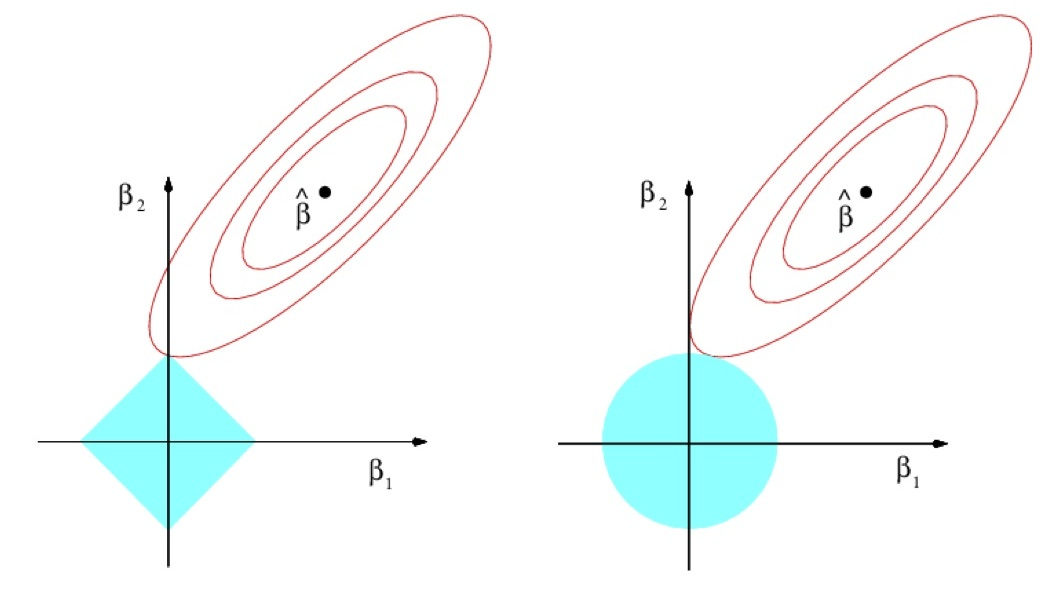In : df_auto = df_auto.assign(kilometrage = lambda r: r.mileage*1.6) df_auto.loc[:, ['mileage', 'kilometrage', 'price']].head()  Out: mileage kilometrage price 0 67697 108315.2 14995 1 73738 117980.8 11988 2 80313 128500.8 11999 3 86096 137753.6 12995 4 79607 127371.2 11333 In : X_train = df_auto.loc[:, ['mileage', 'kilometrage']].values y_train = df_auto.price.values model = Lasso() # model = Ridge() model.fit(X_train, y_train) print('Модель:\nprice = %.2f + (%.2f)*mileage + (%.2f)*kilometrage' % (model.intercept_, model.coef_, model.coef_,)) y_hat = model.predict(X_train) df_auto.plot(x='mileage', y='price', kind='scatter') _ = plt.plot(X_train[:, 0], y_hat, c='r')  Модель: price = 16762.02 + (-0.05)*mileage + (-0.00)*kilometrage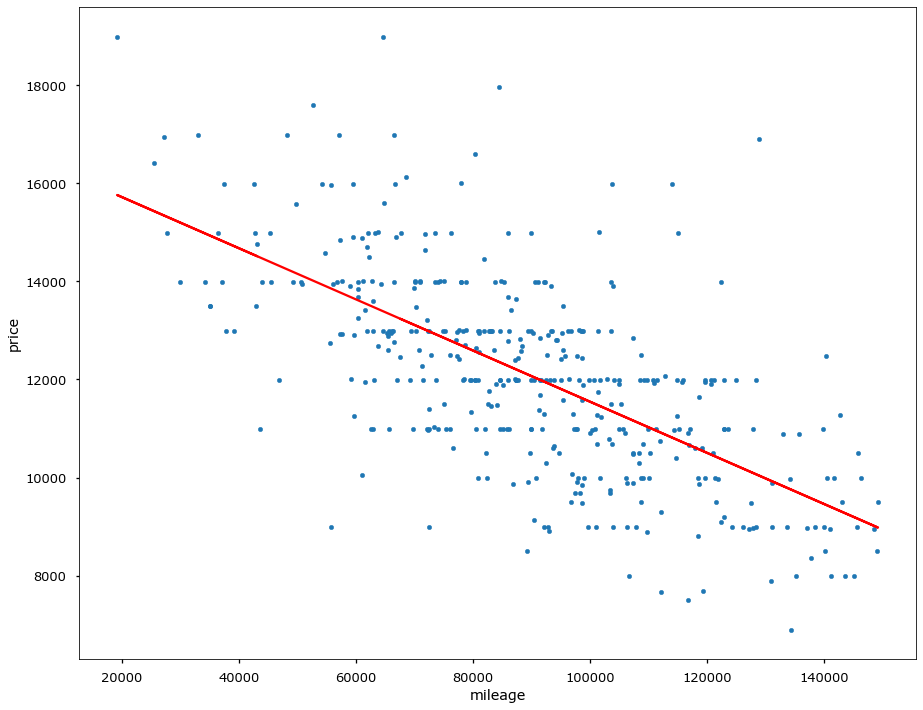## ElasticNet¶ • ElasticNet:$$ R(\beta)=\alpha||\beta||_{1}+(1-\alpha)||\beta||_{2}^{2}\to\min_{\beta} $$\alpha\in(0,1) - hyperparameter, controlling impact of each part. • If two features x^{i}and x^{j} are equal: • LASSO may take only one of them • Ridge will take both with equal weight • but it doesn't remove useless features • ElasticNet both removes useless features but gives equal weight for usefull equal features • good, because feature equality may be due to chance on this particular training set ## Wisdom of the day¶### Overfitting = Death¶ # Linear Classification (in general)¶$$w_1x_1 + w_2x_2 + w_0 = w^{T}x+w_{0}=0 y \in \{-1,+1\}$$• Given weights w and w_0 and feature vector x we can • tell if x is "below" or "above" hyperplane • tell how far is x from hyperplane ## Analytical geometry reminder¶ ## Reminder¶ • a=[a^{1},...a^{D}]^{T},\,b=[b^{1},...b^{D}]^{T} • Scalar product \langle a,b\rangle=a^{T}b=\sum_{d=1}^{D}a_{d}b_{b} • a\perp b means that \langle a,b\rangle=0 • Norm \left\lVert a\right\rVert =\sqrt{\langle a,a\rangle} • Distance \rho(a,b)=\left\lVert a-b\right\rVert =\sqrt{\langle a-b,a-b\rangle}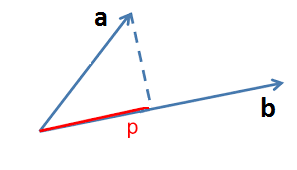• p=\langle a,\frac{b}{\left\lVert b\right\rVert }\rangle • \left|p\right|=\left|\langle a,\frac{b}{\left\lVert b\right\rVert }\rangle\right|- unsigned projection length ## Orthogonal vector to hyperplane¶ ### Theorem 1¶ Vector w is orthogonal to hyperplane w^{T}x+w_{0}=0 Proof: Consider arbitrary x_{A},x_{B}\in\{x:\,w^{T}x+w_{0}=0\}:$$ \begin{align} w^{T}x_{A}+w_{0}=0 \quad \text{ (1)}\\ w^{T}x_{B}+w_{0}=0 \quad \text{ (2)} \end{align} $$By substracting (2) from (1), obtain w^{T}(x_{A}-x_{B})=0, so w is orthogonal to hyperplane.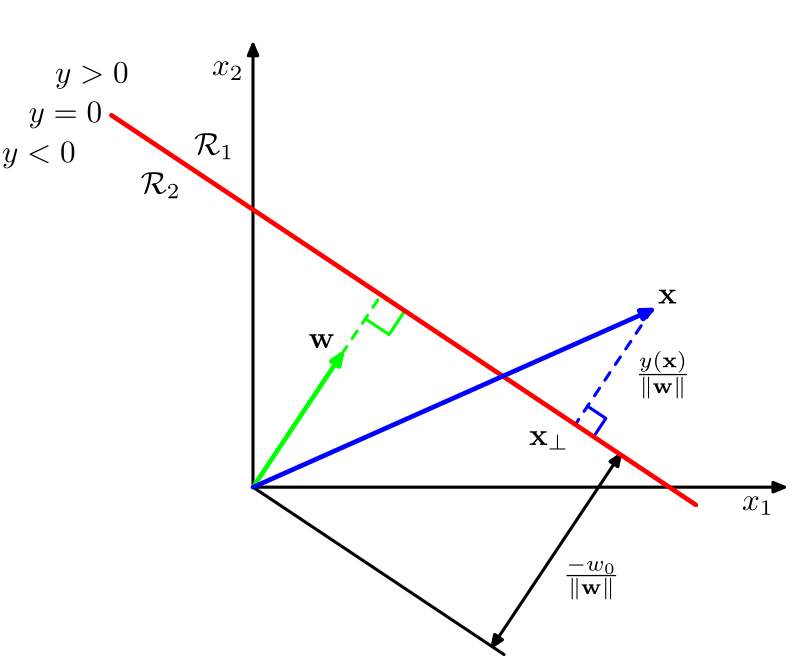## Distance from point to hyperplane¶ ### Theorem 2¶ Distance from point x to hyperplane w^{T}x+w_{0}=0 is equal to \frac{w^{T}x+w_{0}}{\left\lVert w\right\rVert }. Proof: Project x on the hyperplane, let the projection be t and complement h=x-t, orthogonal to hyperplane. Then$$ x=t+h $$Since t lies on the hyperplane,$$ w^{T}t+w_{0}=0 $$Since h is orthogonal to hyperplane and according to theorem 1$$ h=r\frac{w}{\left\lVert w\right\rVert },\,r\in\mathbb{R}\text{ - distance to hyperplane}. $$## Distance from point to hyperplane¶$$ x=t+r\frac{w}{\left\lVert w\right\rVert } $$After multiplication by w and addition of w_{0}:$$ w^{T}x+w_{0}=w^{T}t+w_{0}+r\frac{w^{T}w}{\left\lVert w\right\rVert }=r\left\lVert w\right\rVert $$because w^{T}t+w_{0}=0 and \left\lVert w\right\rVert =\sqrt{w^{T}w}. So we get, that$$ r=\frac{w^{T}x+w_{0}}{\left\lVert w\right\rVert } $$Comments: • From one side of hyperplane r>0\Leftrightarrow w^{T}x+w_{0}>0 • From the other side r<0\Leftrightarrow w^{T}x+w_{0}<0. • Distance from hyperplane to origin 0 is \frac{w_{0}}{\left\lVert w\right\rVert }. So w_{0} accounts for hyperplane offset. ## Binary linear classifier geometric interpretation¶ Binary linear classifier:$$ \widehat{y}(x)= sign\left(w^{T}x+w_{0}\right) $$divides feature space by hyperplane w^{T}x+w_{0}=0. • Confidence of decision is proportional to distance to hyperplane \frac{\left|w^{T}x+w_{0}\right|}{\left\lVert w\right\rVert }. • w^{T}x+w_{0} is the confidence that class is positive. Consider the following objects x1 x2 0 1 1 0 1 1 2 2 2 3 3 2 Find class prediction if (w_0 = -0.3 , w_1 = 0.1, w_2 = 0.1) # Fitting weights¶ ## Margin of binary linear classifier¶$$ M(x,y) =y\cdot\left(w^{T}x+w_{0}\right) $$• y \in \{-1,+1\} • Margin = score, how well classifier predicted true y for object x. • M(x,y|w)>0 \Leftrightarrow object x is correctly classified as y • signs of w^{T}x+w_{0} and y coincide • |M(x,y|w)|=\left|w^{T}x+w_{0}\right| - confidence of decision • proportional to distance from x to hyperplane w^{T}x+w_{0}=0. ## Margin¶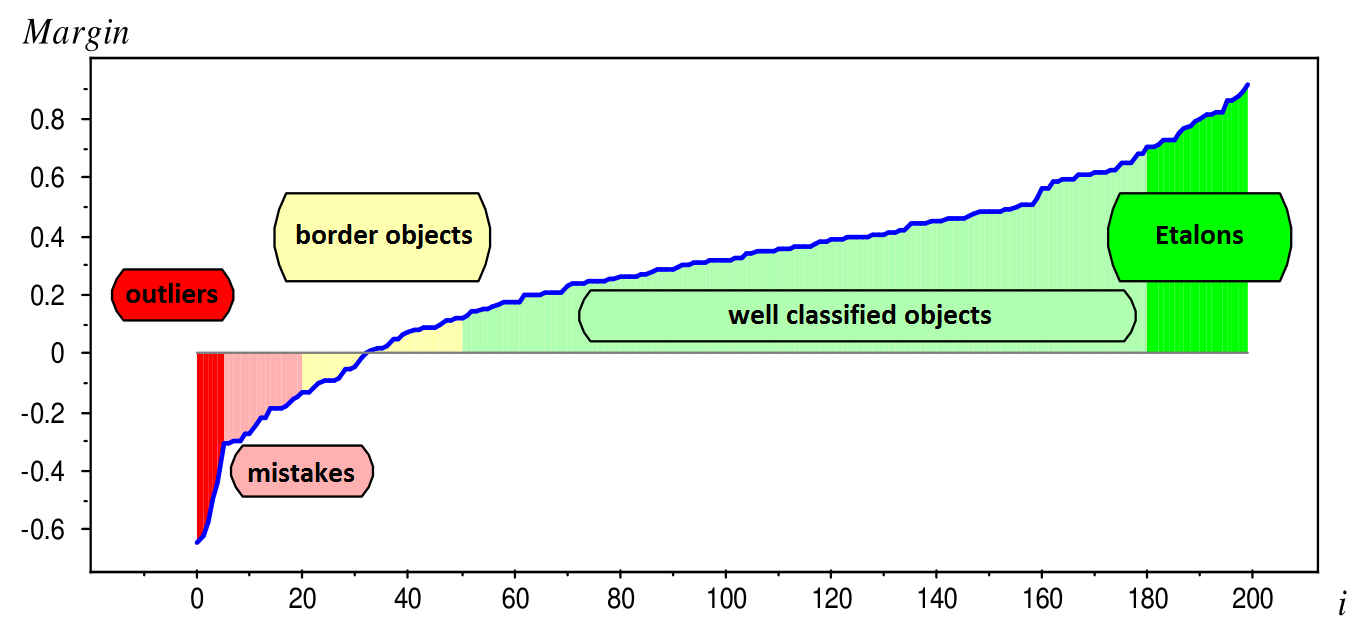## Misclassification rate optimization¶ • Misclassification rate optimization:$$ \frac{1}{N}\sum_{n=1}^{N}\mathbb{I}[M(x_{n},y_{n}|w)<0]\to\min_{w} $$is not recommended: • discontinous function, can't use numerical optimization! • continous margin is more informative than binary error indicator. • If we select loss function \mathcal{L}(M) such that \mathbb{I}[M]\le\mathcal{L}(M) then we can optimize upper bound on misclassification rate:$$ \begin{gathered}\begin{gathered}\text{MISCLASSIFICATION RATE}\end{gathered} =\frac{1}{N}\sum_{n=1}^{N}\mathbb{I}[M(x_{n},y_{n}|w)<0]\\ \le\frac{1}{N}\sum_{n=1}^{N}\mathcal{L}(M(x_{n},y_{n}|w))=L(w) \end{gathered} $$## Optimization problem¶ • Margin = score, how well classifier predicted true y for object x. • Task: select such w to increase M(x_{n},y_{n}|w) for all n. • Formalization:$$ \frac{1}{N}\sum_{n=1}^{N}\mathcal{L}\left(M(x_{n},y_{n}|w)\right)\to\min_{w} $$## Common loss functions¶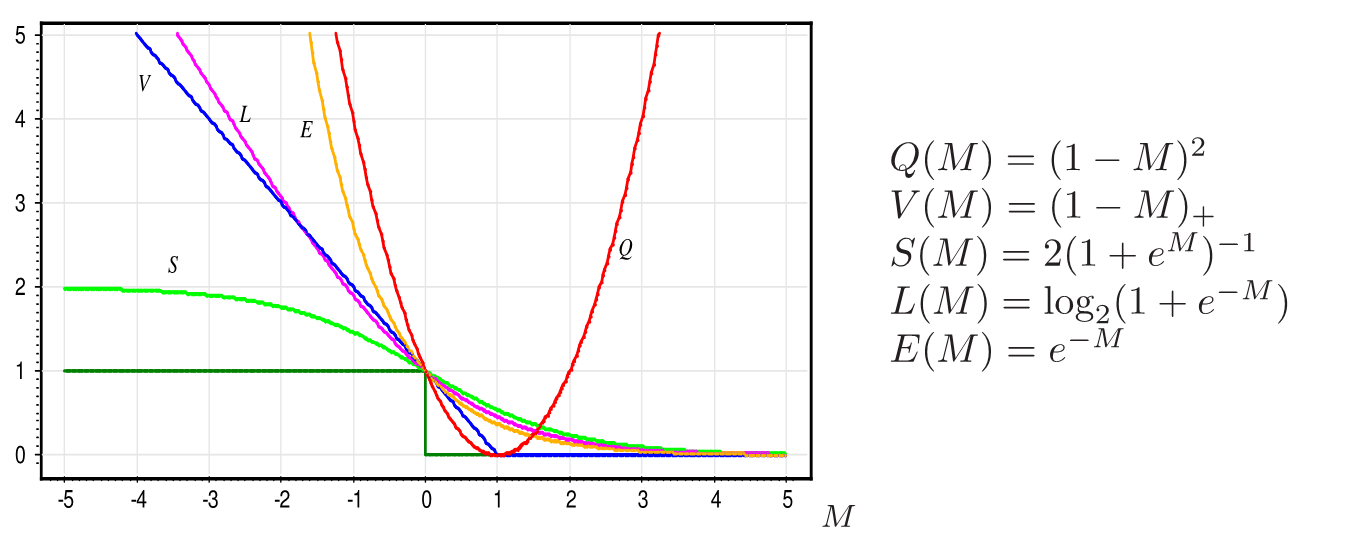# Optimization procedure¶ Same story as in linear regression • analytical solution (if possible) • or gradient descent (most usual case) # Regularization¶ Same story as in linear regression • add regularization terms to initial loss function • question: is it good idea to regularize intercept?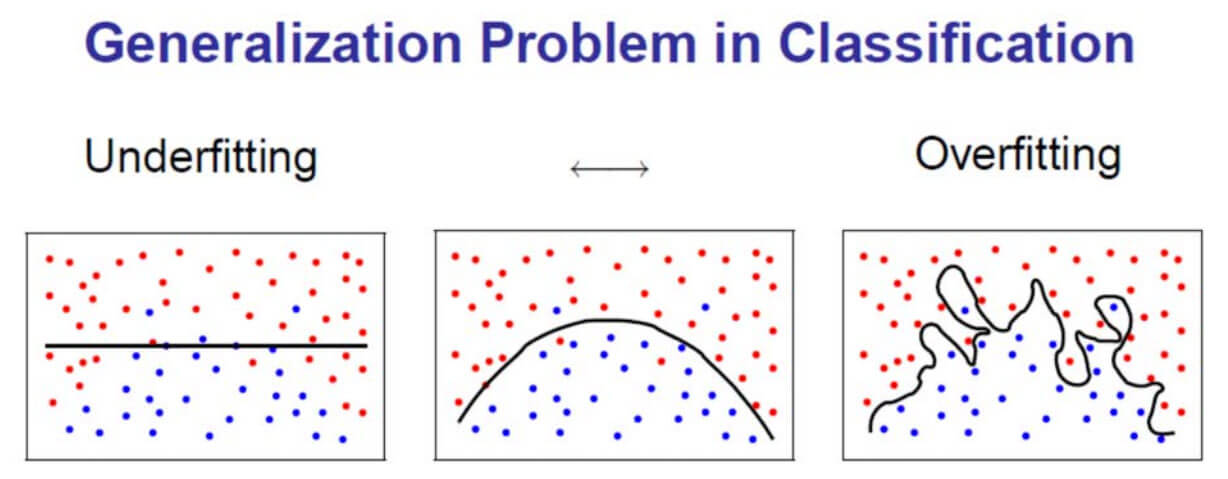## L_{1} regularization¶ • ||w||_{1} regularizer should do feature selection. • Consider$$ L(w)=\sum_{n=1}^{N}\mathcal{L}\left(M(x_{n},y_{n}|w)\right)+\lambda\sum_{d=1}^{D}|w_{d}| $$• And gradient updates$$ \frac{\partial}{\partial w_{i}}L(w)=\sum_{n=1}^{N}\frac{\partial}{\partial w_{i}}\mathcal{L}\left(M(x_{n},y_{n}|w)\right)+\lambda sign (w_{i})  \lambda sign (w_{i})\nrightarrow0\text{ when }w_{i}\to0 $$## L_{2} regularization¶$$ L(w)=\sum_{n=1}^{N}\mathcal{L}\left(M(x_{n},y_{n}|w)\right)+\lambda\sum_{d=1}^{D}w_{d}^{2}  \frac{\partial}{\partial w_{i}}L(w)=\sum_{n=1}^{N}\frac{\partial}{\partial w_{i}}\mathcal{L}\left(M(x_{n},y_{n}|w)\right)+2\lambda w_{i}  2\lambda w_{i}\to0\text{ when }w_{i}\to0 $$• Strength of regularization \to0 as weights \to0. • So L_{2} regularization will not set weights exactly to 0. # Logistic Regression¶ • solves classification tasks #mindblown ## Binary classification¶ • Linear classifier:$$ score(y=+1|x)=w^{T}x + w_0 = g(x) $$• +relationship between score and class probability is assumed:$$ p(y=+1|x)=\sigma(w^{T}x + w_0) $$where \sigma(z)=\frac{1}{1+e^{-z}} - sigmoid function In : demo_sigmoid()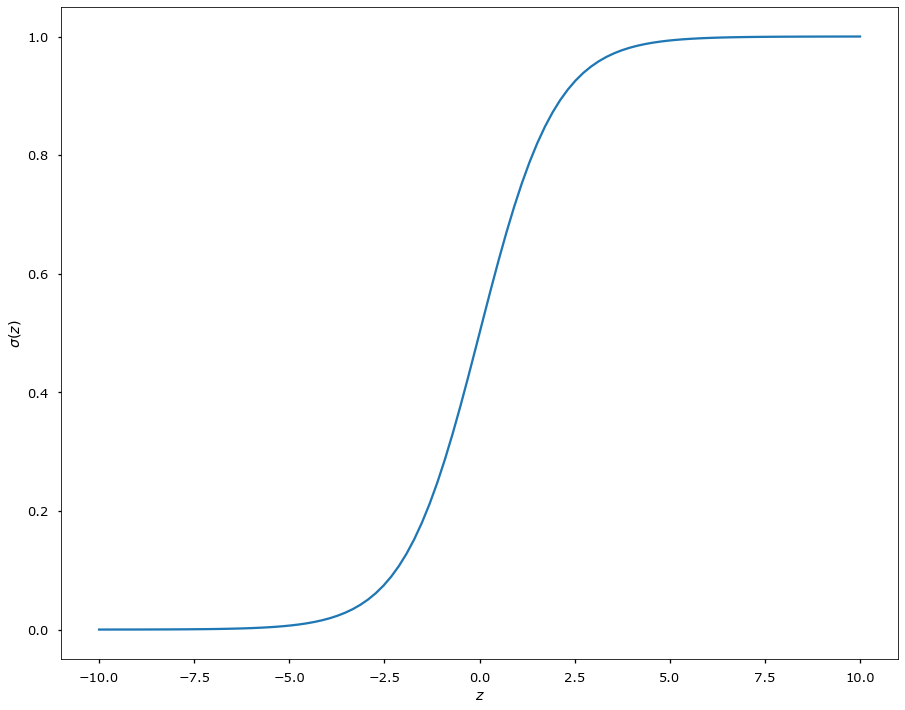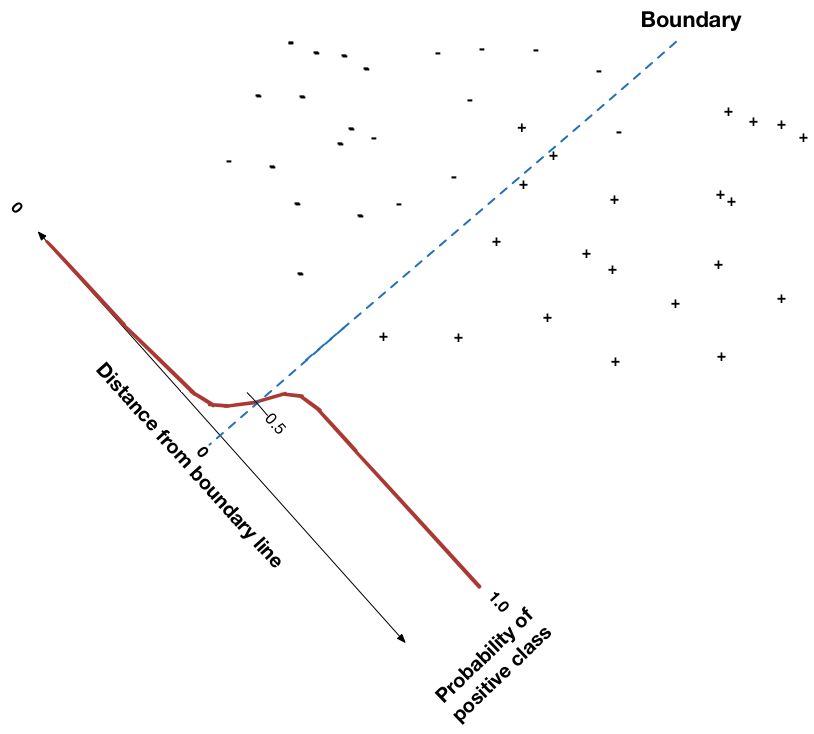## Binary classification: estimation¶ • Given our assumption: • p(y=+1|x)=\sigma(w^{T}x + w_0) • p(y=-1|x)=1 - p(y=+1|x) • we can write down Likelihood function:$$ \mathcal{L}(w) = \prod_{n=1}^N p(y_n=+1|x_n)^{\mathbb{I}[y_n = +1]} p(y_n=-1|x_n)^{\mathbb{I}[y_n = -1]} \rightarrow \max_w$$• Get rid if products:$$ -\ln{\mathcal{L}(w)} = - \sum_{n=1}^N \mathbb{I}[y_n = +1]\cdot\ln{\sigma(w^{T}x_n+w_0))} + \mathbb{I}[y_n = -1]\cdot\ln{(1-\sigma(w^{T}x_n+w_0))} \rightarrow \min_wL(w) = -\ln{\mathcal{L}(w)} \rightarrow \min_w $$• Function L(w) is also called log-loss In : plot_logloss()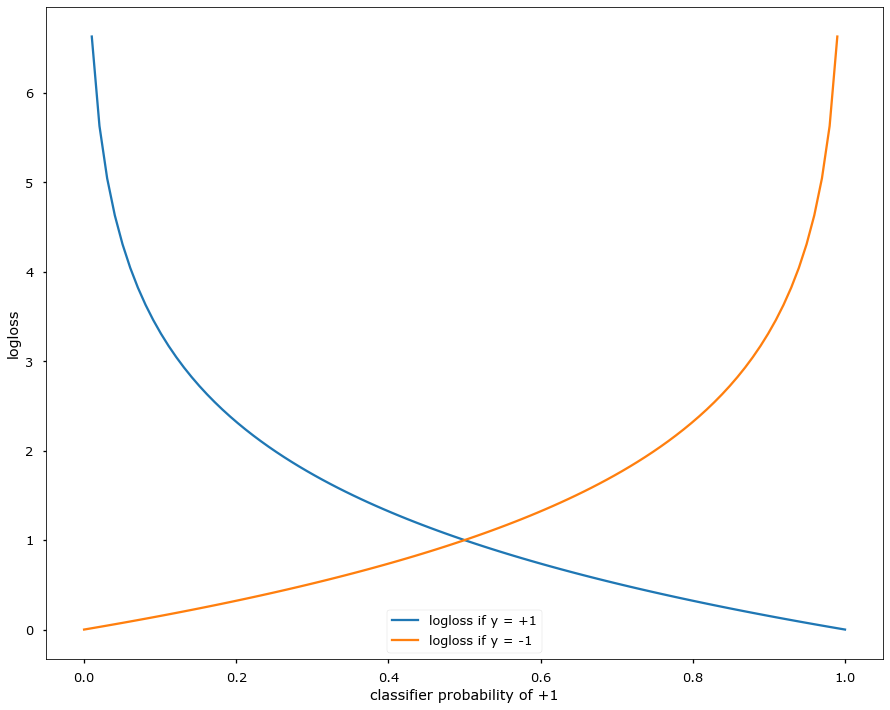In : _ = interact(linedist_demo, w1=FloatSlider(min=-5, max=5, value=1., step=0.5), w2=FloatSlider(min=-5, max=5, value=-1., step=0.51), w0=FloatSlider(min=-5, max=5, value=0., step=0.5))  ## Another formulation with Margin¶ Using the property 1-\sigma(z)=\sigma(-z) obtain that$$ p(y=+1|x)=\sigma(w^{T}x+w_0)\Longrightarrow p(y=-1|x)=\sigma(-w^{T}x - w_0) $$So for y\in\{+1,-1\}$$ p(y|x)=\sigma(y(\langle w,x\rangle + w_0)) $$"probability of correct classification" Therefore ML estimation can be written as:$$ \prod_{n=1}^{N}\sigma( y_{n}(\langle w,x_{n}\rangle + w_0))\to\max_{w} $$## Loss function for binary logistic regression¶ For binary classification p(y|x)=\sigma(y(\langle w,x\rangle + w_0)) Estimation with ML:$$ \prod_{n=1}^{N}\sigma(y_n(\langle w,x_n\rangle + w_0)) = \prod_{n=1}^{N}\sigma(y_n g(x_n)) \to\max_{w} $$which is equivalent to$$ \sum_{n=1}^{N}\ln(1+e^{-y_ng(x_n)})\to\min_{w} $$It follows that logistic regression is linear discriminant estimated with loss function \mathcal{L}(M)=\ln(1+e^{-M}).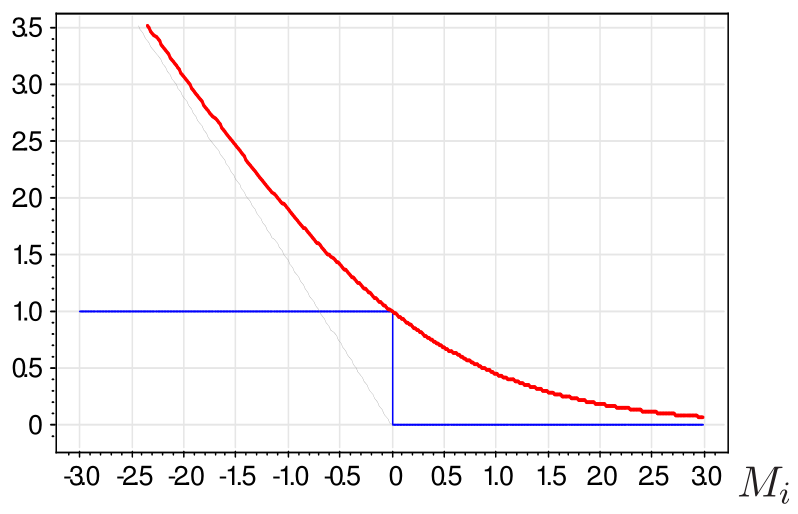# Multiclass classification with binary classifiers¶ ## Multiclass classification with binary classifiers¶ • Task - make C-class classification using many binary classifiers. • Approaches: • one-versus-all • for each c=1,2,...C train binary classifier on all objects and output \mathbb{I}[y_{n}=c], • assign class, getting the highest score in resulting C classifiers. • one-versus-one • for each i,j\in[1,2,...C], i\ne j learn on objects with y_{n}\in\{i,j\} with output y_{n} • assign class, getting the highest score in resulting C(C-1)/2 classifiers. ## Binary linear classifier¶ • For two classes y\in\{+1,-1\} classifier becomes$$ \widehat{y}(x)=\begin{cases} +1, & w_{+1}^{T}x+w_{+1,0}>w_{-1}^{T}x+w_{-1,0}\\ -1 & \text{otherwise} \end{cases} $$• This decision rule is equivalent to$$ \begin{align*} \widehat{y}(x) & =sign(w_{+1}^{T}x+w_{+1,0}-w_{-1}^{T}x+w_{-1,0})=\\ & =sign\left(\left(w_{+1}^{T}-w_{-1}^{T}\right)x+\left(w_{+1,0}-w_{-1,0}\right)\right)\\ & =sign\left(w^{T}x+w_{0}\right) \end{align*} $$for w=w_{+1}-w_{-1},\,w_{0}=w_{+1,0}-w_{-1,0}. • Decision boundary w^{T}x+w_{0}=0 is linear. • Multiclass case can be solved using multiple binary classifiers with one-vs-all, one-vs-one • can you imagine faulty situation with those approaches for linear classifiers?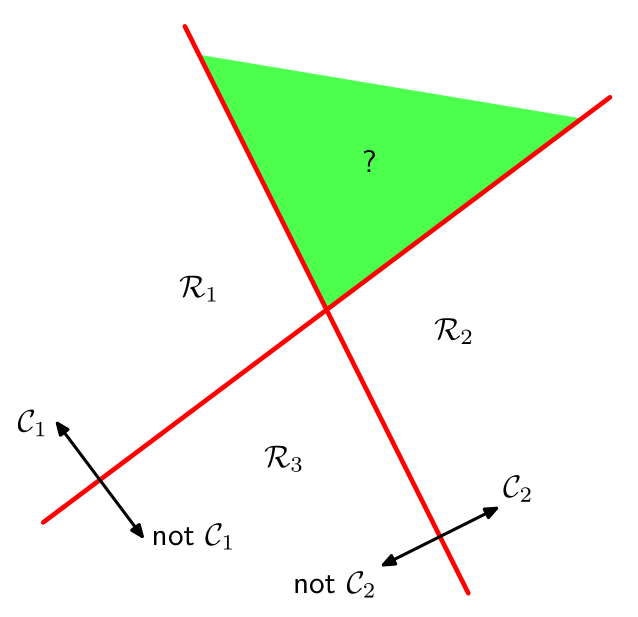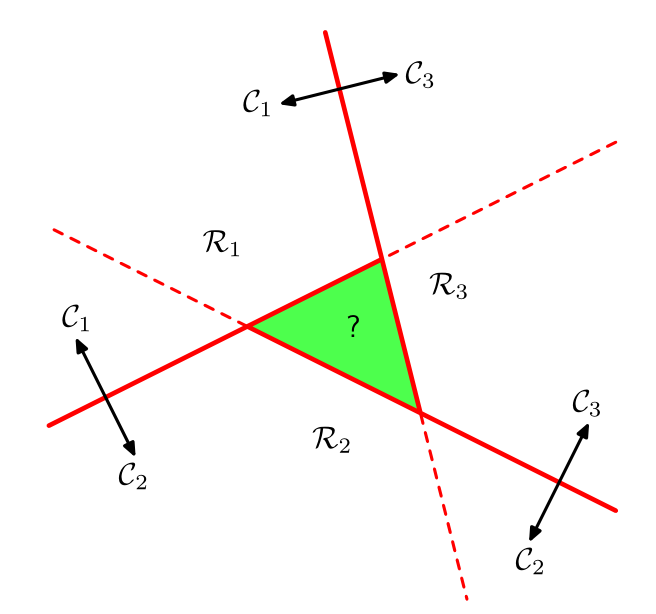## Linear classifier for multiple classes¶ • Classification among classes 1,2,\dots,C. • Use C discriminant functions g_{c}(x)=w_{c}^{T}x+w_{c0} • Decision rule:$$ \widehat{y}(x)=\arg\max_{c}g_{c}(x) $$• Decision boundary between classes y=i and y=j is linear:$$ \left(w_{i}-w_{j}\right)^{T}x+\left(w_{i0}-w_{j0}\right)=0 $$• Decision regions are convex ## Logistic regression for multiple classes¶ Each class has a set of weights:$$ \begin{cases} score(\omega_{1}|x)=w_{1}^{T}x + w_{0,1} \\ score(\omega_{2}|x)=w_{2}^{T}x + w_{0,2}\\ \cdots\\ score(\omega_{C}|x)=w_{C}^{T}x + + w_{0,C} \end{cases} $$+relationship between score and class probability is assumed:$$ p(\omega_{c}|x)=softmax(\omega_c|W, x)=\frac{exp(w_{c}^{T}x + w_{0,c})}{\sum_{i}exp(w_{i}^{T}x + w_{0,i})} $$Estimation with ML:$$ \prod_{n=1}^{N}\prod_{c=1}^{C} softmax(\omega_c|W, x_n)^{\mathbb{I}[y_n = w_c]} $$Which would lead us to cross-entropy loss function$$L(w) = - \sum_{n=1}^N\sum_{c=1}^{C} \mathbb{I}[y_n = w_c]\cdot\ln{\sigma(w_c^{T}x_n+w_{c,0}))}$## Summary¶ • Linear classifier - classifier with linear discriminant functions. • Binary linear classifier:$\widehat{y}(x)=sign(w^{T}x+w_{0})$. • Perceptron, logistic, SVM - linear classifiers estimated with different loss functions. • Weights are selected to minimize total loss on margins. • Regularization gives smooth control over model complexity. •$L_{1}\$ regularization automatically selects features.
• Elements of Statistical Learning (Trevor Hastie, Robert Tibshirani, Jerome Friedman) - Chapter 4.4
• Pattern Recognition and Machine Learning (Christopher M. Bishop) - Chapter 4